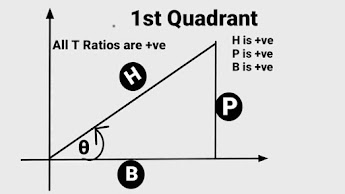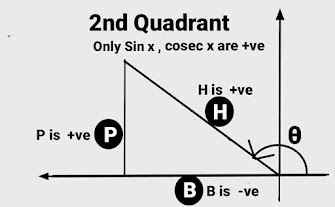reasoning (43) Maths (40) Home (8) integration (6)

## HOW TO MEMORISE DIFFERENT VALUES OF TRIGONOMETRIC ANGLES

Welcome to this post of learning trigonometric formulas in an easy way with me. Because most of the Students or Mathematics Learner ,most of the time confuse to remember or memorise value of different trigonometric angles in different quadrants and could not reproduce what they have learnt . So today we are  going to learn new techniques to learn "How to memorise different values of trigonometric angles in various quadrants" forever. Before this we must have knowledge of different trigonometric values of different angles in different quadrants.

## When angle lies in 1st Quadrant

(1) When angle lies in 1st quadrant, then all the t- Ratios have positive values. As in 1st quadrant all the three  sides Perpendicular ,base and hypotenuse of  right angled triangle are positive.

(2) When angle involve  π/2 ,then T - Ratios on the left hand side  will changes accordingly , if  t-Ratios start with "co" , then co will be removed and if it is  without "co" then co will be added at the beginning .i.e. sin x will changes to cos x, tan  x to cot x , cos x to sin x ,cosec c to sec x and sec  x to cosec x.MEMORISING TRIGONOMETRIC ANGLES

Table for  Trigonometric Angles  in 1st Quadrant
S
N

Trigonometric

Angles
Value of T  Angles
1 Sin (π/2 - x)   cos x
2 Cos (π/2 - x)   sin x
3 tan (π/2 - x)   cot x
4 Cot (π/2 - x)    tan x
5 Sec (π/2 - x)    Cosec x
6 Cosec (π/2 - x)     Sec x

(3)  When angle involve  2π ,then T - Ratios on the left hand side  will NOT changes , put the same  t -ratios which is on the left hand side on right hand side i.e.  sin x  to sin x  , cos x  to cos x  , tan x  to tan  x and so on.

Table for  Trigonometric Angles  in 1st Quadrant
S
N

Trigonometric

Angles
Value of T  Angles
1 Sin (2π + x)  Sin x
2 Cos (2π + x)  cos x
3tan (2π + x)   tan x
4Cot (2π + x)   Cot x
5Sec (2π + x)   Sec x
6Cosec (2π + x)    Cosec x

## When angle lies in 2nd  Quadrant

(1)  when angle lies in 2nd quadrants ,then only two t - ratios sin x and it reciprocal cosec x shall have +ve values and remaining t-Ratios shall have -ve values. As in 2nd  quadrant two  out of the three  sides Perpendicular and  hypotenuse of  right angled triangle are positive  while base is negative. So in all those  t -ratios ,when base involves  they will be negative. So  cos x, tan x ,cot x ,sec x involve with -ve value of base therefore these t- ratios shall be negative.

(2)  When angle involve  π/2 ,then T - Ratios on the left hand side  will changes accordingly , if  t-Ratios start with "co" , then co will be removed and if it is  without "co" then co will be added at the beginning .i.e. sin x will changes to cos x, tan x to cot x and sec x to cosec x.MEMORISING TRIGONOMETRIC ANGLES

Table for  Trigonometric Angles  in 2nd Quadrant
S
N

Trigonometric

Angles
Value of T  Angles
1 Sin (π/2 + x)   cos x
2 Cos (π/2 + x)   -sin x
3 tan (π/2 + x)   -cot x
4 Cot (π/2 + x)    -tan x
5 Sec (π/2 + x)    cosec x
6 Cosec (π/2 + x)     sec x

(3) When angle  involve  π ,then T - Ratios on the left hand side will NOT changes , put the same  t -ratios which is on the left hand side on right hand side i.e.  sin x to sin x , cos x to cos x , tan x to tan x  and so on.

Table for  Trigonometric Angles  in 2nd Quadrant
S
N

Trigonometric

Angles
Value of T  Angles
1 Sin (π - x)   Sin x
2 Cos (π - x)   - cos x
3 tan (π - x)    -tan x
4 Cot (π - x)    -Cot x
5 Sec (π - x)    -Sec x
6 Cosec (π - x)     Cosec x

## When angle lies in 3rd Quadrant

(1)  when angle lies in 3rd quadrants ,then only two t - ratios tan x and it reciprocal cot x shall have +ve values and remaining t-Ratios shall have -ve values. As in 3rd  quadrant two  out of the three  sides perpendicular and  base of  right angled triangle are negative  and hypotenuse is positive. So in all those  t -ratios ,when one of perpendicular or base  involves  they will be negative. So  sin x, cos x , sec x ,cosec x involve with -ve value of base or perpendicular therefore these t- ratios shall be negative. And tan x and cot x involves with both -ve values of perpendicular and base so they are positive in 3rd quadrant.

(2)  When angle involve  π/2 ,then T - Ratios on the left hand side  will changes accordingly , if  t-Ratios start with "co" , then co will be removed and if it is  without "co" then co will be added at the beginning .i.e. sin x will changes to cos x, tan x to cot x and sec x  to cosec x.

Table for  Trigonometric Angles  in 3rd Quadrant

S
N

Trigonometric

Angles
Value of T  Angles
1 Sin (3π/2 - x)   -Cos x
2 Cos (3π/2 - x)   -Sin x
3 tan (3π/2 - x)    cot x
4 Cot (3π/2 - x)    tan x
5 Sec (3π/2 - x)    -Cosec x
6 Cosec (3π/2 - x)    -Sec x

(3)  When angle involve  π ,then T - Ratios on the left hand side  will NOT changes , put the same  t -ratios which is on the left hand side on right hand side i.e.  sin x to sin x , cos x to cos x , tan x to tan x  and so on.

Table for  Trigonometric Angles  in 3rd Quadrant
S
N

Trigonometric

Angles
Value of T  Angles
1 Sin (π + x)   -Sin x
2 Cos (π + x)   - cos x
3 tan (π + x)    tan x
4 Cot (π + x)    Cot x
5 Sec (π + x)    -Sec x
6 Cosec (π + x)     -Cosec x

Let us understand these learning of trigonometric formulae with the help of this video

## When angle lies in 4th Quadrant

(1)  when angle lies in 4th quadrants ,then only two t - ratios cos x and it reciprocal sec x shall have +ve values and remaining t-Ratios shall have -ve values. As in 4th quadrant two out of the three sides Base and hypotenuse of right angled triangle are positive and perpendicular is negative. So in all those t -ratios ,when perpendicular involves they will be negative. So sin x, tan x ,cot x ,cosec x involve with -ve value of perpendicular therefore these t- ratios shall be negative.

(2)  When angle involve  π/2 ,then T - Ratios on the left hand side  will changes accordingly , if  t-Ratios start with "co" , then co will be removed and if it is  without "co" then co will be added at the beginning .i.e. sin x will changes to cos x, tan x to cot x and sec x  to cosec x.

Table for  Trigonometric Angles  in 4th Quadrant

S
N

Trigonometric

Angles
Value of T  Angles
1 Sin (3π/2 + x)   -Cos x
2 Cos (3π/2 + x)   Sin x
3 tan (3π/2 + x)    -cot x
4 Cot (3π/2 + x)    -tan x
5 Sec (3π/2 + x)    Cosec x
6 Cosec (3π/2 + x)    -Sec x

So if we want to calculate sin 300° ,sin 240° and sin 330°  then it can be find out as follows

sin 300° = sin (270° + 30° ) = - cos 30° = -√3/2
sin 330° = sin (360° - 30° )  = - sin 30° = -1/2
sin 240° = sin (270° - 30° ) = - cos 30° = -√3/2

and if we want to calculate cos 300° , cos 240° and   cos 330°  then it can be find out as follows
cos 300° = cos (270° + 30° ) =  sin 30° = 1/2
cos 330° = cos (360° - 30° )  =  cos 30° = √3/2
cos 240° = cos (270° - 30° ) = - sin 30° = 1/2

(3)  When angle involve  π ,then T - Ratios on the left hand side  will NOT changes , put the same  t -ratios which is on the left hand side on right hand side i.e.  sin x to sin x , cos x to cos x , tan x to tan x  and so on.

Table for  Trigonometric Angles  in 4th Quadrant

S
N

Trigonometric

Angles
Value of T  Angles
1 Sin (2π - x)   -Sin x
2 Cos (2π - x)   cos x
3 tan (2π - x)    -tan x
4 Cot (2π - x)    -Cot x
5 Sec (2π - x)    Sec x
6 Cosec (2π - x)     -Cosec x

### When Angle do not involve π

Table for  Trigonometric Angles  in 4th Quadrant
S
N

Trigonometric

Angles
Value of T  Angles
1 Sin (- x)   -Sin x
2 Cos (- x)   cos x
3 tan (- x)   -tan x
4 Cot (- x)    -Cot x
5 Sec (- x)    Sec x
6 Cosec (- x)     -Cosec x

## GENERALISATION OF THE FORMULAE

So when an angle involves integral multiple of π , i,e -3π, -2π, -π, 2π, 3π, 4π then of the T-Ratios will change , But + or - sign can be added at beginning , e. g . sin(nπ + x) may change to + sin x or - sin x ,similarly cos (nπ + x) may change to + or - cos x depending upon the quadrant in which angle lies.

So if we want to find the value of sin 1110° ,then it can be written as sin (3×360° + 30°) = sin 30° = 1/2 . (As the angle is lying in 1st Quadrant )

Similarly if we want to find the value of sin 1050° ,then it can be written as sin (3×360° - 30°) = - sin 30° = - 1/2. (As the angle is lying in 4th Quadrant )

and when an angle involves odd integral multiple of π/2 i.e. (2n+1)π/2 , i . e -7π/2 , -5π/2 , -3π/2 , π/2 , 3π/2 , 5π/2.

## Conclusion

Thanks for devoting your valuable time for the post memorising different values of trigonometric angles in different quadrants of this blog. If you found this  blog/post of your concern, Do Follow me on my blog and share  post with your friends . We shall meet again in next post ,till then Good Bye.

Share:

#### 1 comment:

1.nice post..thank you for sharing

Your valuable suggestions are always acceptable to us for betterment of this website